# Chemical Equilibrium MCQ - 1 (Advanced)

## 25 Questions MCQ Test Chemistry for JEE Advanced | Chemical Equilibrium MCQ - 1 (Advanced)

Description
Attempt Chemical Equilibrium MCQ - 1 (Advanced) | 25 questions in 60 minutes | Mock test for JEE preparation | Free important questions MCQ to study Chemistry for JEE Advanced for JEE Exam | Download free PDF with solutions
*Multiple options can be correct
QUESTION: 1

### Which of the following statements is (are) correct ?

Solution:
*Multiple options can be correct
QUESTION: 2

### The value of equilibrium constant of a reversible reaction at a given temperature :

Solution: Equilibrium constant is independent of original concentration of reactant.
*Multiple options can be correct
QUESTION: 3

### Which of the following statement is (are) correct ?

Solution:
*Multiple options can be correct
QUESTION: 4

If is found that the equilibrium constant increases by factor of four when the temperature is increased from 25°C to 40°C. The value of ΔH° is

Solution:

As we know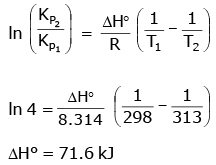*Multiple options can be correct
QUESTION: 5

Ammonium carbamate dissociates as follows :

NH2COONH4(s)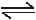2NH3(g) + CO2(g)

The value of KP for this reaction is found to be equal to 2.92 × 10-5 atm3. If one mole of ammonium carbamate is heated in a sealed container, the total pressur develped in the container is

Solution: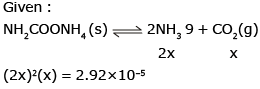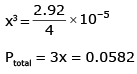*Multiple options can be correct
QUESTION: 6

The equilibrium constant for the following reactions at 1400 K are given.

2H2O(g)2H2(g) + O2(g) ; K1=2.1×10-13

2CO2(g)2CO(g)+O2(g);K2 = 1.4 × 10-12

Then, the equilibrium constant K for the reaction

H2(g) + CO2(g)CO(g) + H2O(g) is

Solution: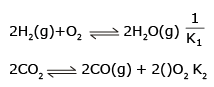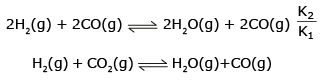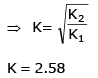*Multiple options can be correct
QUESTION: 7

Under what conditions of temperature and pressure, the formation of atomic hydrogen from molecular hydrogen will be favoured most ?

Solution: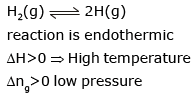*Multiple options can be correct
QUESTION: 8

For the reaction PCl5(g)PCl3(g) + Cl2(g), the forward reaction at constant temperature is favoured by

Solution:

Introduction of inet gas at constant Pressure will increase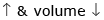Pressure of gases of equilibrium
⇒ equilibrium shifted forward

*Multiple options can be correct
QUESTION: 9

When NaNO3(s) is heated in a closed vessel, oxygen is liberated and NaNO2(s) is left behind. At equilibrium.

Solution: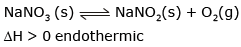High temperature
∴  forward reaction favoured 1000 pressure
High pressure → Reverse reaction

*Multiple options can be correct
QUESTION: 10

Phase diagram of CO2 is shown as following

Based on above find the correct statement (s)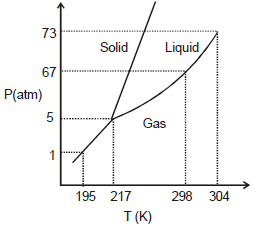Solution:
*Multiple options can be correct
QUESTION: 11

The equilibrium between, gaseous isomers A, B and C can be represented as

Reaction                                           Equilibrium constant

A(g)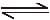B (g)                            : K1 = ?

B(g)C(g)                             : K2 = 0.4

C(g)A(g)                             : K3 = 0.6

If one mole of A is taken in a closed vessel of volume 1 litre, then

Solution:
*Multiple options can be correct
QUESTION: 12

For the gas phase exothermic reaction.

A2 + B2C2, carried out in a closed vessel, the equilibrium moles of A2 can be increased by

Solution:

According to Le Chatelier’s Principle A, B and C are correct.

Hence A, B and C

*Multiple options can be correct
QUESTION: 13

Consider the equilibrium

HgO(s) + 4I- (aq) + H2O(l)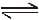Hg I42- (aq) + 2OH- (aq), which changes will decrease the equilibrium concentration of HgI42-

Solution:

As given :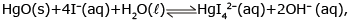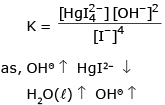*Multiple options can be correct
QUESTION: 14

In the laboratory the equilibrium constant for a particular reaction can be measured at different temperatures. Plotting the data yields the graph shown. Which of the following statements is false?

(Note : The notation 4.40 e- 4 is equivalent to 4.40 × 10-4)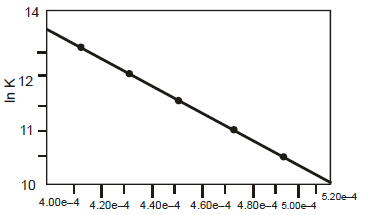Solution: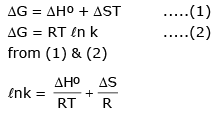slope of the line equal to ΔHº/R

*Multiple options can be correct
QUESTION: 15

Decrease in the pressure for the following equilibria : H2O (s)H2O(l) result in the :

Solution: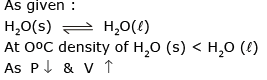∴ formation of more H2O (s) and  increase in melting point of H2O (s)

QUESTION: 16

Statement-1 : Water boils at high temperature in pressure cooker.

Statement-2 : Increase in pressure leads to an increase in boiling point.

Solution:

Statement-1 is true, statement-2 is true and statement-2 is correct explanation for statement-1

QUESTION: 17

Statement-1 : A decrease in pressure leads to an increase in freezing point of water.

Statement-2 : For ice on melting volume decreases.

Solution:

Statement-1 is true, statement-2 is true and statement-2 is correct explanation for statement-1

QUESTION: 18

Statement-1 : The solubility of gases always increases with increase in pressure.

Statement-2 : High pressure favours the change where volume of gas decreases.

Solution:

Statement-1 is true, statement-2 is true and statement-2 is correct explanation for statement-1

QUESTION: 19

Statement-1 : Total number of moles in a closed system at new equilibrium is less than the old equilibrium if some amount of a substance is removed from a system.

A(g)B(g) equilibrium.

Statement-2 : The number of moles of the substance which is removed, is partially compensated as the system reached to new equilibrium.

Solution:

Statement-1 is true, statement-2 is true and statement-2 is NOT the correct explanation for statement-1

QUESTION: 20

Statement-1 : An exothermic reaction, non-spontaneous at high temperature, may become spontaneous at low temperature.

Statement-2 : Entropy of an exothermic reaction always decrease with decrease in temperature.

Solution:

Statement-I is false, statement-2 is true.

QUESTION: 21

Statement-1 : Ammonia at a pressure of 10 atm and CO2 at a pressure of 20 atm are intoduced into an evacuated chamber. If Kp for the reaction.

NH2COONH4(s)2NH3(g) + CO2(g) is 2020 atm3, the total pressure after a long time is less than 30 atm.

Statement-2 : Equilibrium can be attained from both directions.

Solution:

Statement-1 is true, statement-2 is false.

QUESTION: 22

In a 7.0 L evacuated chamber, 0.50 mol H2 and 0.50 mol I2 react at 427°C

H2(g) + I2 (g)2HI(g). At the given temperature, Kc = 49 for the reaction.

What is the value of Kp ?

Solution: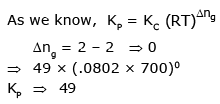QUESTION: 23

In a 7.0 L evacuated chamber, 0.50 mol H2 and 0.50 mol I2 react at 427°C

H2(g) + I2 (g)2HI(g). At the given temperature, Kc = 49 for the reaction.

What is the total pressure (atm) in the chamber

Solution: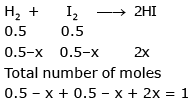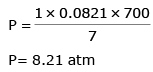QUESTION: 24

In a 7.0 L evacuated chamber, 0.50 mol H2 and 0.50 mol I2 react at 427°C

H2(g) + I2 (g)2HI(g). At the given temperature, Kc = 49 for the reaction.

How many moles of the iodine remain unreacted at equilibrium ?

Solution:

from above question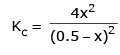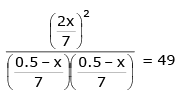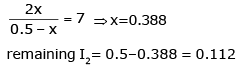QUESTION: 25

In a 7.0 L evacuated chamber, 0.50 mol H2 and 0.50 mol I2 react at 427°C

H2(g) + I2 (g)2HI(g). At the given temperature, Kc = 49 for the reaction.

What is the partial pressure (atm) of HI in the equilibrium mixture ?

Solution: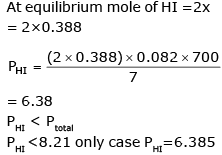Use Code STAYHOME200 and get INR 200 additional OFF Use Coupon Code